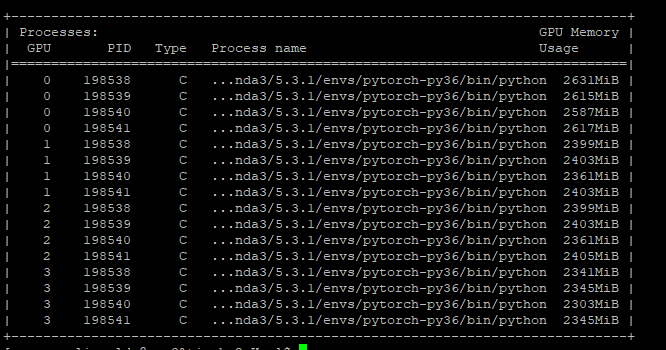# There are many processes have been created on each node when I use DDP package

Today I want to use DISTRIBUTED COMMUNICATION PACKAGE to train the imagenet, however I found there are many processes have been created on the node.

script.py:

``````def main():
...
model.to(device)
model = torch.nn.parallel.DistributedDataParallel(model, device_ids=None, output_device=None, find_unused_parameters=True)
...

if __name__ == '__main__':
print('Use {back} as backend.'.format(back=args.backend))
dist.init_process_group(backend=args.backend, init_method='env://', timeout=datetime.timedelta(seconds=1000))
main()

``````

bash.sh:

``````CUDA_VISIBLE_DEVICES=0,1,2,3
export GLOO_SOCKET_IFNAME=ib0
export OMP_NUM_THREADS=24
NPROC_PER_NODE=4
SLURM_JOB_NUM_NODES=4
...

COMMAND="script.py -a inception_v3 --print-freq 1000 --backend gloo --nproc-per-node 4 --pretrained  --multiprocessing-distributed  \$HOME/ImageNet"

python -m torch.distributed.launch \
--nproc_per_node=\$NPROC_PER_NODE \
--nnodes=\$SLURM_JOB_NUM_NODES \
--node_rank=\$SLURM_NODEID \
--master_addr=\$MIP \
--master_port=\$MPORT \
\$COMMAND > \$HOME/thesis/PCL/log/"log_v1_inception_"\${SLURM_JOB_ID}"_"\${SLURM_NODEID}".out"
``````

I have trained it in 4 nodes and there are 4 gpus on each node.

I log in the node0 then I found there are 16 processes in this nodeIs it abnormal? Or DDP would create nproc_per_nodee * node_num processes on each node?

Hey @khalil, sorry about the delay. Looking at the screenshot, they are actually the same set of processes, but each process used all GPUs. Any reason for setting `device_ids` to `None`, instead of `device_ids=[local_rank]`? If it is None, by default, DDP will use all visible CUDA devices.